Note: This is an archvied version of our old webpage. Some links might be broken. The current one can be found here.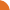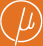Chair for Foundations of Software Reliability and Theoretical Computer Science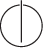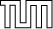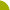Publications - On Computing the Total Variation Distance of Hidden Markov ModelsReference:

Stefan Kiefer. On computing the total variation distance of hidden Markov models. Technical report, arXiv.org, 2018. Available at https://arxiv.org/abs/1804.06170.

Abstract:

We prove results on the decidability and complexity of computing the total variation distance (equivalently, the L1-distance) of hidden Markov models (equivalently, labelled Markov chains). This distance measures the difference between the distributions on words that two hidden Markov models induce. The main results are: (1) it is undecidable whether the distance is greater than a given threshold; (2) approximation is #P-hard and in PSPACE.

Suggested BibTeX entry:

@techreport{18K-ICALP-TR,
author = {Stefan Kiefer},
institution = {arXiv.org},
note = {Available at https://arxiv.org/abs/1804.06170},
title = {On Computing the Total Variation Distance of Hidden {M}arkov Models},
year = {2018}
}See arxiv.org ...Conference version